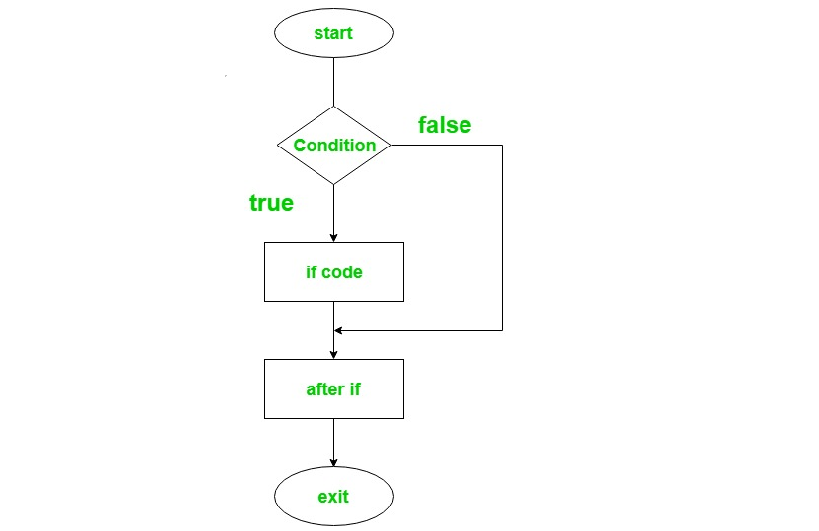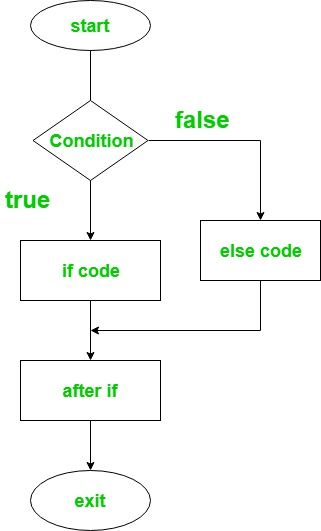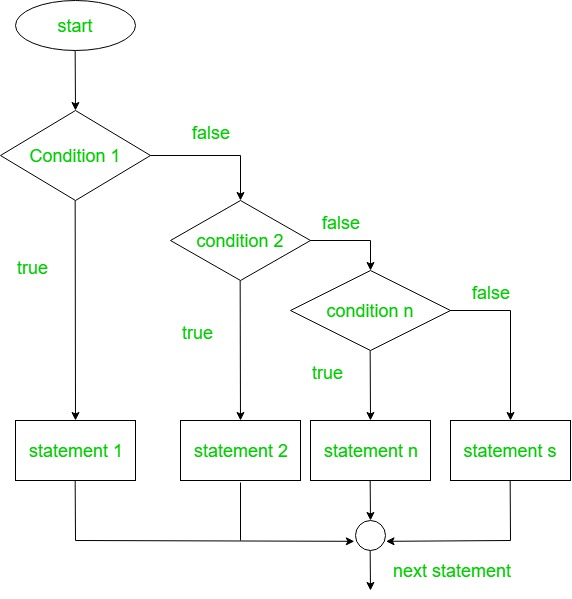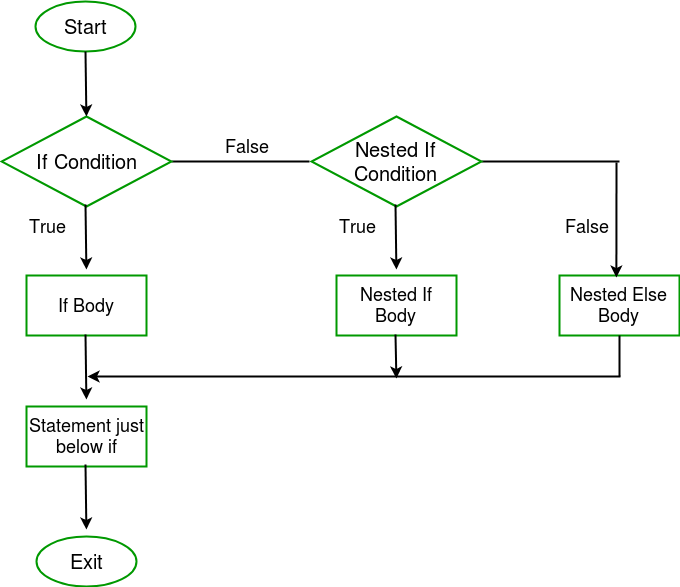# Kotlin if-else expression

Decision Making in programming is similar to decision making in real life. In programming too, a certain block of code needs to be executed when some condition is fulfilled.A programming language uses control statements to control the flow of execution of program based on certain conditions.If condition is true then it enters into the conditional block and executes the instructions.

There are different types of if-else expressions in Kotlin:

• if expression
• if-else expression
• nested if expression

if statement:
It is used to specify a block of statements to be executed or not i.e if a certain condition is true then the statement or block of statements to be executed otherwise fails to execute.
Syntax:

```if(condition) {

// code to run if condition is true
}
```

Flowchart:Example:

 `fun main(args: Array) { ` `    ``var a = ``3` `    ``if``(a > ``0``){ ` `        ``print(``"Yes,number is positive"``) ` `    ``} ` `} `

Output:

`Yes, number is positive`

if-else statement:
if-else statement contains two blocks of statements. ‘if’ statement is used to execute the block of code when the condition becomes true and ‘else’ statement is used to execute a block of code when the condition becomes false.
Syntax:

``` if(condition) {
// code to run if condition is true
}
else {
// code to run if condition is false
}
```

Flowchart:Here is the Kotlin program to find the larger value of two numbers.

 `fun main(args: Array) { ` `        ``var a = ``5` `        ``var b = ``10` `        ``if``(a > b){ ` `            ``print(``"Number 5 is larger than 10"``) ` `        ``} ` `        ``else``{ ` `            ``println(``"Number 10 is larger than 5"``) ` `        ``} ` `    ``} `

Output:

`Number 10 is larger than 5`

Kotlin if-else expression as ternary operator –
In Kotlin, if-else can be used as an expression because it returns a value. Unlike java, there is no ternary operator in Kotlin because if-else return the value according to the condition and works exactly similar to ternary.

Below is the Koltin program to find the greater value between two numbers using if-else expression.

 `fun main(args: Array) { ` `    ``var a = ``50` `    ``var b = ``40` ` `  `    ``// here if-else returns a value which  ` `    ``// is to be stored in max variable ` `    ``var max = ``if``(a > b){                   ` `        ``print(``"Greater number is: "``) ` `        ``a ` `    ``} ` `    ``else``{ ` `        ``print(``"Greater number is:"``) ` `        ``b ` `    ``} ` `    ``print(max) ` `} `

Output:

`Greater number is: 50`

Here, a user can put multiple conditions. All the ‘if’ statements are executed from the top to bottom. One by one all the conditions are checked and if any of the condition found to be true then the code associated with the if statement will be executed and all other statements bypassed to the end of the block. If none of the conditions is true, then by default the final else statement will be executed.
Syntax:

```if(Firstcondition) {
// code to run if condition is true
}
else if(Secondcondition) {
// code to run if condition is true
}
else{
}
```

Flowchart:Below is the Kotlin program to determine the number is positive, negative or equal to zero.

 `import` `java.util.Scanner ` ` `  `fun main(args: Array) { ` `    `  `    ``// create an object for scanner class ` `    ``val reader = Scanner(System.`in`)        ` `    ``print(``"Enter any number: "``) ` ` `  `    ``// read the next Integer value ` `    ``var num = reader.nextInt()              ` `    ``var result  = ``if` `( num > ``0``){ ` `        ``"\$num is positive number"` `    ``} ` `    ``else` `if``( num < ``0``){ ` `        ``"\$num is negative number"` `    ``} ` `    ``else``{ ` `        ``"\$num is equal to zero"` `    ``} ` `    ``println(result) ` ` `  `} `

Output:

```Enter any number: 12
12 is positive number

Enter any number: -11
-11 is negative number

Enter any number: 0
0 is zero
```

nested if expression:
Nested if statements means an if statement inside another if statement.If first condition is true then code the associated block to be executed, and again check for the if condition nested in the first block and if it is also true then execute the code associated with it. It will go on until the last condition is true.
Syntax:

```if(condition1){
// code 1
if(condition2){
// code2
}
}
```

Flowchart:Below is the Kotlin program to determine the largest value among three Integers.

 `import` `java.util.Scanner ` ` `  `fun main(args: Array) { ` `  `  `    ``// create an object for scanner class ` `    ``val reader = Scanner(System.`in`)        ` `    ``print(``"Enter three numbers: "``) ` ` `  `    ``var num1 = reader.nextInt() ` `    ``var num2 = reader.nextInt() ` `    ``var num3 = reader.nextInt() ` ` `  `    ``var max  = ``if` `( num1 > num2) { ` `        ``if` `(num1 > num3) { ` `            ``"\$num1 is the largest number"` `        ``} ` `        ``else` `{ ` `            ``"\$num3 is the largest number"` `        ``} ` `    ``} ` `    ``else` `if``( num2 > num3){ ` `        ``"\$num2 is the largest number"` `    ``} ` `    ``else``{ ` `        ``"\$num3 is the largest number"` `    ``} ` `    ``println(max) ` ` `  `} `

Output:

```Enter three numbers: 123 231 321
321 is the largest number
```

My Personal Notes arrow_drop_upCheck out this Author's contributed articles.

If you like GeeksforGeeks and would like to contribute, you can also write an article using contribute.geeksforgeeks.org or mail your article to contribute@geeksforgeeks.org. See your article appearing on the GeeksforGeeks main page and help other Geeks.

Please Improve this article if you find anything incorrect by clicking on the "Improve Article" button below.

Improved By : Akanksha_Rai

Article Tags :

Be the First to upvote.

Please write to us at contribute@geeksforgeeks.org to report any issue with the above content.